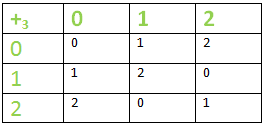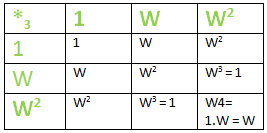Related Articles

# Homomorphism & Isomorphism of Group

• Last Updated : 26 May, 2021

Introduction :
We can say that  “o” is the binary operation on set G if : G is an non-empty set & G * G = { (a,b) : a , b∈ G } and  o : G * G –> G. Here, aob denotes the image of ordered pair (a,b) under the function / operation o.
Example – “+” is called a binary operation on G (any non-empty set ) if & only if : a+b ∈G ; ∀ a,b ∈G and a+b give the same result every time when added.
Real example – ‘+’ is a binary operation on the set of natural numbers ‘N’ because a+b ∈ N ; ∀ a,b ∈N and a+b  a+b give the same result every time when added.

Laws of Binary Operation :
In a binary operation o, such that : o : G * G –> G on the set G is :
1. Commutative –

` aob = boa ; ∀ a,b ∈G`

Example :  ‘+’ is a binary operation on the set of natural numbers ‘N’. Taking any 2 random natural numbers , say 6 & 70, so here a = 6 & b = 70,
a+b = 6 + 70 = 76 = 70 + 6 = b + a
This is true for all the numbers that come under the natural number.

2. Associative –

`ao(boc) = (aob)oc ; ∀ a,b,c ∈G`

Example :  ‘+’ is a binary operation on the set of natural numbers ‘N’. Taking any 3 random natural numbers , say 2 , 3 & 7, so here a = 2 & b = 3 and c = 7,
LHS : a+(b+c) = 2 +( 3 +7)  = 2 + 10 = 12
RHS : (a+b)+c = (2 + 3) + 7 = 5 + 7 = 12
This is true for all the numbers that come under the natural number.

3. Left Distributive –

`ao(b*c) = (aob) * (aoc) ; ∀ a,b,c ∈G`

4. Right Distributive –

` (b*c) oa = (boa) * (coa)  ; ∀ a,b,c ∈G`

5. Left Cancellation –

` aob =aoc  => b = c  ; ∀ a,b,c ∈G`

6. Right Cancellation –

` boa = coa  => b = c ; ∀ a,b,c ∈G`

Algebraic Structure :
A non-empty set G equipped with 1/more binary operations is called an algebraic structure.
Example : a. (N,+)  and b. (R, + , .), where N is a set of natural numbers & R is a set of real numbers. Here ‘ . ‘ (dot) specifies a multiplication operation.

GROUP :
An algebraic structure (G , o) where G is a non-empty set & ‘o’ is a binary operation defined on G is called a Group if the binary operation “o” satisfies the following properties –

1. Closure –

`a ∈ G ,b ∈ G  => aob ∈ G ;  ∀ a,b ∈ G`

2. Associativity –

` (aob)oc = ao(boc) ; ∀ a,b,c ∈ G.`

3. Identity Element –
There exists e in G such that aoe = eoa = a ; ∀ a ∈ G (Example – For addition, identity is 0)

4. Existence of Inverse –
For each element a ∈ G ; there exists an inverse(a-1)such that :  ∈ G such that – aoa-1 = a-1oa = e

Homomorphism of groups :
Let (G,o) & (G’,o’) be 2 groups, a mapping “f ” from a group (G,o) to a group (G’,o’) is said to be a homomorphism if –

`f(aob) = f(a) o' f(b) ∀ a,b ∈ G`

The essential point here is : The mapping f : G –> G’ may neither be a one-one nor onto mapping, i.e, ‘f’ needs not to be bijective.

Example –
If (R,+) is a group of all real numbers under the operation ‘+’ & (R -{0},*) is another group of non-zero real numbers under the operation ‘*’ (Multiplication) &  f is a mapping from (R,+) to (R -{0},*),  defined as : f(a) = 2a ;  ∀ a ∈ R
Then f is a homomorphism like –  f(a+b) = 2a+b = 2a * 2b = f(a).f(b) .
So the rule of homomorphism is satisfied & hence f is a homomorphism.

Homomorphism Into –
A mapping ‘f’, that is homomorphism & also Into.

Homomorphism Onto
A mapping ‘f’, that is homomorphism & also onto.

Isomorphism of Group :
Let (G,o) & (G’,o’) be 2 groups, a mapping “f ” from a group (G,o) to a group (G’,o’) is said to be an isomorphism if –

```1. f(aob) = f(a) o' f(b) ∀ a,b ∈ G
2. f is a one- one mapping
3. f is an onto mapping.```

If ‘f’ is an isomorphic mapping, (G,o) will be isomorphic to the group (G’,o’) & we write :

`G ≅ G'`

Note : A mapping f: X -> Y is called :

1. One – One  – If x1 ≠x2, then f(x1) ≠ f(x2) or if f(x1) = f(x2) => x1 = x2. Where x1,x2 ∈ X
2. Onto           – If every element in the set Y is the f-image of at least one element of set X.
3. Bijective      – If it is one one & Onto.

Example of Isomorphism Group –
If G is the multiplicative group of 3 cube-root units , i.e., (G,o) = ( {1, w, w2 } , *) where w3 = 1 &  G’ is an additive group of integers modulo 3 –  (G’, o’) = ( {1,2,3) , +3). Then : G ≅ G’ , we say G is isomorphic to G’.• The structure & order of both  the tables are same. The mapping ‘f’ is defined as :
f : G -> G’ in such a way that f(1) = 0 , f(w) = 1 & f(w2) = 2.
• Homomorphism property : f(aob) = f(a) o’ f(b) ∀ a,b ∈ G . Let us take a = w & b = 1
LHS : f(a * b) = f( w * 1 ) = f(w) = 1.
RHS : f(a) +3 f(b) = f(w) +3 f(1) = 1 + 0 = 1
=>LHS = RHS
• This mapping f is one-one & onto also, therefore, a homomorphism.

Attention reader! Don’t stop learning now.  Practice GATE exam well before the actual exam with the subject-wise and overall quizzes available in GATE Test Series Course.

Learn all GATE CS concepts with Free Live Classes on our youtube channel.

My Personal Notes arrow_drop_up